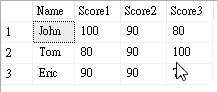### [SQL][Quiz]找出欄位中最大值

``````SELECT * INTO #Students
FROM ( VALUES ( 'John' , 100, 90 , 80 ),( 'Tom' , 80, 90 , 100 ),( 'Eric' , 90, 90 , 70 ) ) Students( Name, Score1,Score2,Score3)
GO

SELECT * FROM #Students
GO``````1. 使用 CASE 的作法 ( 如果要判斷的欄位不多是還算簡單 )

``````SELECT Name,
CASE
WHEN ( Score1 >= Score2 ) AND ( Score1 >= Score3 ) THEN Score1
WHEN ( Score2 >= Score1 ) AND ( Score2 >= Score3 ) THEN Score2
WHEN ( Score3 >= Score1 ) AND ( Score3 >= Score2 ) THEN Score3
END AS MaxScore
FROM #Students``````

2. 在 SELECT 使用 VALUES 來轉換，我比較喜歡的做法，但可能有些人不習慣這樣的寫法 )

``````SELECT Name ,
( SELECT Max(score)
FROM ( Values (Score1),(Score2),(Score3)) T(Score) ) AS MaxScore
FROM #Students``````

3. 利用 UNION 將資料切分多筆，人工作 UNPIVOT 的處理

``````SELECT Name, Max(Score) MaxScore FROM
(
SELECT Name, Score1 AS Score
FROM #Students
UNION
SELECT Name, Score2
FROM #Students
UNION
SELECT Name, Score3
FROM #Students

) Students(Name, Score)
GROUP BY Name``````

4. 跟 3 的做法觀念相同，但改用 UNPIVOT 的指令來處理

``````SELECT Name, MAX(SCORE) AS MaxScore
FROM #Students
UNPIVOT ( SCORE FOR DateVal IN ( Score1, Score2, Score3 ) ) AS ScoreTable
GROUP BY Name``````